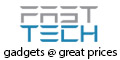## OHM’S LAW Explained

When calculating or examining any electrical circuit three factors must be taken into account;

Current

Voltage

Resistance

These three units of measurement are always intimately interconnected and any combination of the three dictates the overall make-up, or electrical output of an electrical circuit.

Current :  A unit of Current is called an Ampere and is represented by the letter I.

Voltage :  A unit of Voltage is called a Volt and is represented by the letter V.

Resistance :  A unit of Resistance is called an Ohm and is represented by the letter R.

Current (I) is the movement of electrically charged particles. Current is expressed in AmperesAn Ampere is the fundamental unit of current, usually simply expressed colloquially as anAmp and is a quantity representing the intensity of the charge.

Voltage (V) is electromotive force.  Voltage is expressed in VoltsIt is also known as Potential Difference, which is the difference of electrical potential between two points. 1 Volt is the difference of potential which will carry 1 Ampere of Current against 1 Ohm of resistance.

Resistance (R) is expressed as Ohms. Resistance inhibits Current flow.  If Resistance in an electrical circuit were to increase, Current flow will decrease and vice-versa. One Ohm, or unit of Resistance allows the transmission of one Ampere of Current when subjected to the potential difference of 1 Volt.

The inter-relationship of these three integral electrical elements is simply and succinctly explained in Ohm’s Law.  OHM’S LAW (not to be mistaken for OHMS – On Her Majesty’s Service!) was devised in the 1800’s by German Physicist G.S. Ohm.

OHM’S LAW;

### “Current is proportional to Voltage and inversely proportional to Resistance.”

Simply Explained:  “INVERSELY PROPORTIONAL” means the inverted ratio or relation between two quantities; as one increases, the other decreases.  “PROPORTIONAL” means corresponding in degree or amount; if one quantity increases, the other increases by an equal increment.

Current INVERSELY PROPORTIONAL to Resistance If Resistance goes up, Current goes down,  If Resistance goes down, Current goes up.

Current PROPORTIONAL to Voltage If Current goes up, Voltage goes up.  If current goes down, Voltage goes down.

NB:   We can say too that by extension, Voltage is clearly also inversely proportional to Resistance.

Ohm’s Law can be expressed as one of several equations:  V = IR  or  I = V/R  or  R = V/I

These equations can be placed in a pie chart or remembered simply as V=IxR.  If you can remember this equation written in this way;

V
___
I    R

you will be able to quickly and easily calculate the Resistance, Current and Voltage of any electrical circuit when provided with two out of three of these factors.  Whichever factor is missing, simply place your thumb over it and calculate the other two.

To whit;

VOLTAGETo calculate Voltage (V) place your thumb over the V as this is the missing element.  Now you see that Voltage equals I x R I (current) is multiplied by R (Resistance).

CURRENTTo calculate Current (I) place your thumb over the symbol representing current (I).  You will now see that Current equals V/R, Voltage divided by Resistance.

RESISTANCE. To calculate Resistance (R) place your thumb over the R, representing Resistance. You can see that Resistance equals V/I, which is Voltage divided by Current.